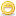CodeGuru Home VC++ / MFC / C++ .NET / C# Visual Basic VB Forums Developer.com

# Thread: [RESOLVED] Decimal cin-Input confusion

1.Junior MemberJoin Date
Jan 2010
Posts
2

## [RESOLVED] Decimal cin-Input confusion

Ok so im new to c++, in fact im pretty new to programming so yeah bear with my lack of knowledge, im writing a basic math test program to show a uni at an interview that I can create simple console programs using c++. It involves the program asking the question, the user answering, if the user input via cin is equal to the equation stored in the integer then correct move onto next question, if not, try the question again, you have 3 lives then the program closes and you must restart and try again.

So its all good so far for example question 1:

int result;

//Question 1
std:: cout << "----------------------------" << std:: endl;
std:: cout << "-Question 1:\n" << std:: endl;

do
{
std:: cout << "What is the result of 35 + 47? ";
std:: cin >> result;
if (lives == 0)
{
std:: cout << "\n\tSorry, you have no more lives.. exiting program\n" << std:: endl;
return 0;
}
else if (result == 35 + 47)
{
std:: cin.get(); std:: cin.get();
break;
}
else
{
lives -= 1;
std:: cout << "\n\tIncorrect. You have " << lives << " More Lives."
" Please Try Again.\n" << std:: endl;
}
} while (result != 35 + 47);

---------------------------------------------------------------------------------------------------------------------

Works fine.

So I thought id create a money question to put some variety in there:

---------------------------------------------------------------------------------------------------------------------

float money_question;

//Question 6
std:: cout << "----------------------------" << std:: endl;
std:: cout << "-Question 6:\n" << std:: endl;

do
{
std:: cout << "If John buys 4 carrots each the price of 24 pence, and 2 onions at the "
"price of 67 pence each with a 5 pound note, how much change will he get? ";
std:: cin >> money_question;
if (lives == 0)
{
std:: cout << "\n\tSorry, you have no more lives.. exiting program\n" << std:: endl;
return 0;
}
else if (money_question == 5.00 - (0.24 * 4) - (0.67 * 2))
{
std:: cin.get(); std:: cin.get();
break;
}
else
{
lives -= 1;
std:: cout << "\n\tIncorrect. You have " << lives << " More Lives."
" Please Try Again.\n" << std:: endl;
}
} while (money_question != 5.00 - (0.24 * 4) - (0.67 * 2));

Now my problem is that the program will say that the cin input 2.70 is incorrect :S

I appreciate any help, sorry about the long post btw, i always write too much..Reply With Quote

2.MemberJoin Date
Jun 2005
Posts
315

## Re: Decimal cin-Input confusion

While I haven't fully read your post, I did see your use of floats. Not sure but maybe this FAQ will help.Reply With Quote

3.Junior MemberJoin Date
Jan 2010
Posts
2

##Re: [RESOLVED] Decimal cin-Input confusion

Thanks alot, from what i read in your link float numbers are never precise, so my program was waiting for a precise number and never getting it, so i changed it to a double and it works fine nowReply With Quote

4.MemberJoin Date
Jun 2005
Posts
315

## Re: [RESOLVED] Decimal cin-Input confusion

Good news!Reply With Quote

c++, decimal####Posting Permissions

• You may not post new threads
• You may not post replies
• You may not post attachments
• You may not edit your posts
•